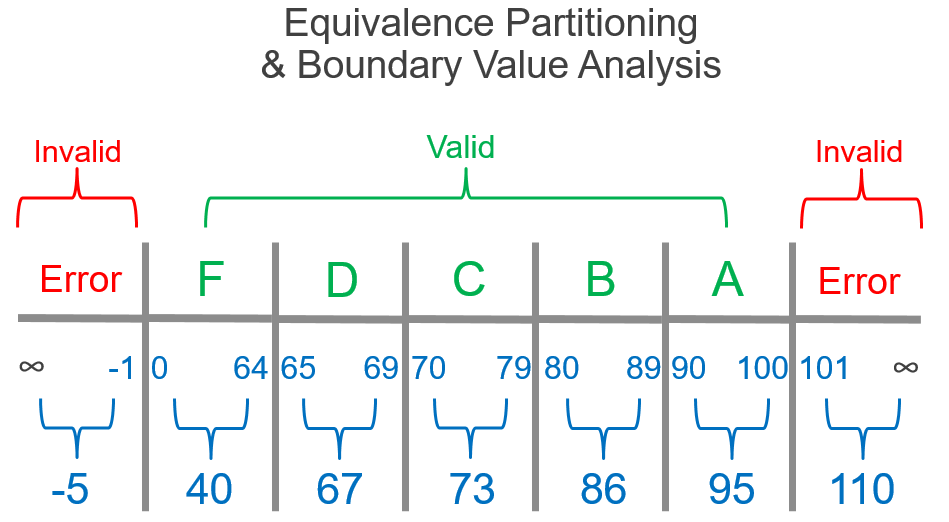# Chapter 6 Testing Frameworks

## 6.1 What exactly is a test

Testing is just checking that the expected result is the same as the actual result. Automated testing is moving from doing this in a informal ad-hoc way to an automated process which is repeatable.

Tests should be

• Fast
• Independent
• Repeatable (deterministic)
• Self-validating (no manual steps)
• Thorough (How much do you trust they cover everything?)

There are common testing frameworks which make testing much simpler.

## 6.2 Unit Test

Unit testing is the cornerstone of modern software development. The purpose is to validate that each unit of the software performs as designed. A unit is the smallest testable part, such as a function. A unit test might check that for a given input you get a certain output. This gives assurance that for the tested cases your function will preform as expected. This might be checking that your code preforms as expected when given a missing value, or a negative value. If all tests are passing then all of the cases that are tested for will work. This removes a lot of the burden of review. Unit testing increases confidence in maintaining or changing code. This is because a test which catch if a change has introduced an error quickly. This also encourages good practice as to make unit testing possible code will need to be modular making it easier to reuse.

## 6.3 Integration Tests

Unit tests ensure that the units of code works as expected, integration tests ensure that the units work together. An example is testing to ensure that a series of functions together do what is expected. In R and Python this can be achieved using the same framework as unit tests. There is a question about how much of your code should you test. This depends on the complexity of system and the importance. For statistics QAAD can be used as framework to gauge the appropriate level of testing. For modeling the AQUA book gives a framework for the appropriate level of QA.

## 6.4 What to tests

A QA engineer walks into a bar. Orders a beer. Orders 0 beers. Orders 99999999999 beers. Orders a lizard. Orders -1 beers. Orders a ueicbksjdhd…

What tests should be written to ensure that your code is preform as expected will vary depending on need, output and specific circumstances. There are some general principles to testing which will cover alot of common issues.

• Usual Data types
• Checking that code works with expected data but also unexpected data types. Common checks would be for positive/negative values, missing values (NA/NaN), 0, very large numbers, words when there should be numbers and many more. This might involve checking that when given certain values the code doesn’t do something, such as produce a value when given an illogical input.
• Application Program Interface, API
• This involves testing the interface to the code, which is where the user will interact with the code. As you are unable to control what they input this is a common source of errors.
• Output
• For a given input, what is the function expected to return such as a data table, a list, a number etc. What kind of data structure the function is supposed to return (to make sure other functions work, if it expects a specific data structure).
• Boundary Value Analysis & Equivalence Partitioning
• This is an approach to reduce the total number of test cases to a finite set of testable test cases. It is a useful approach to making testing a structured method rather than a series of ad-hoc tests which may not cover important sources of error. Boundary analysis involves testing the extreme ends or boundaries between partitions of the input values. Equivalence Partitioning divides the input data into different equivalence data classes which can each be tested covering the full spectrum of possible values. An example is illustrative. The chart below shows an example of what to test for student grades, with certain values falling into grade classes, such as 70-79 being awarded a C grade. It would be time consuming to test every number so a Boundary Value Analysis & Equivalence Partitioning approach is taken. We want to test that a number in the grade category returns that award and that values below 0 or above 100 are invalid. This reduces the number of tests which need to be written to a pragmatic and proportionate level.